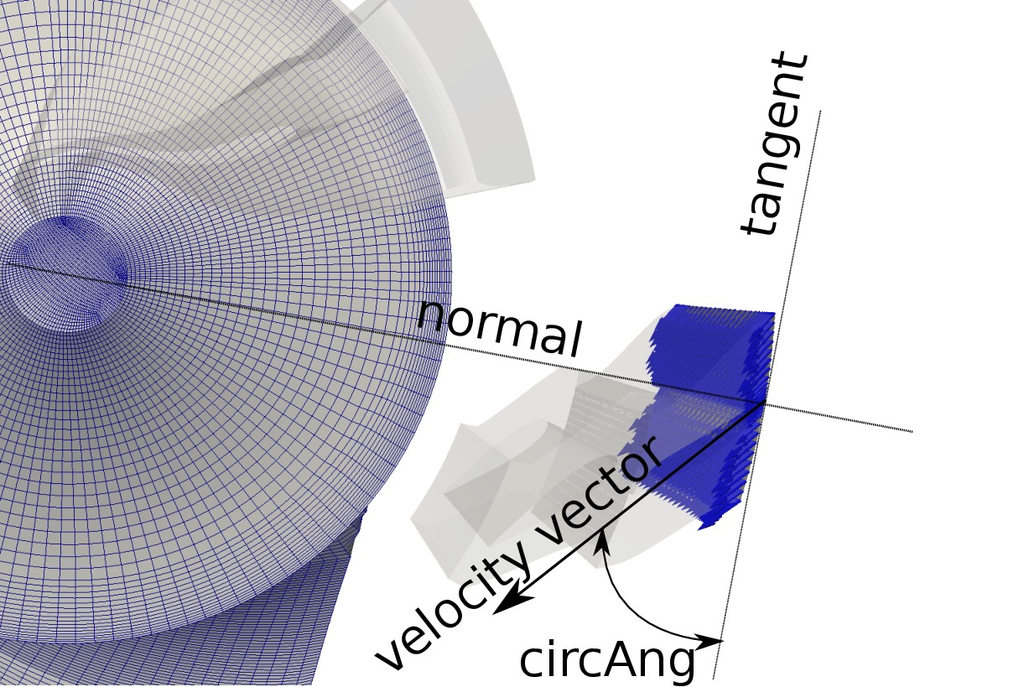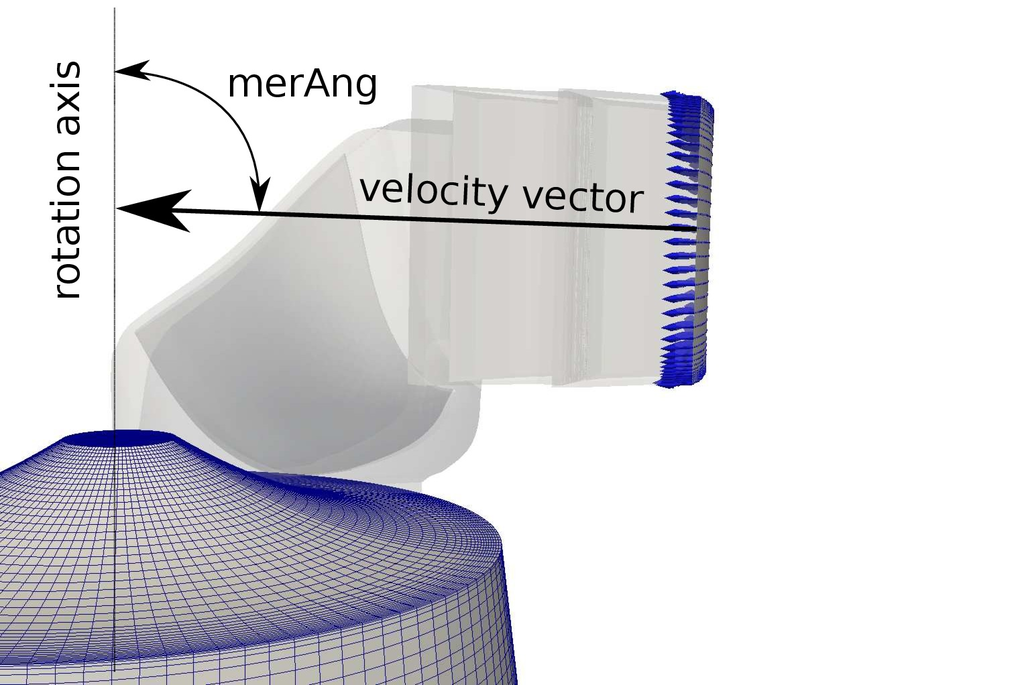## Total Pressure and Velocity Angles Boundary Condition

In many cases it is useful to prescribe total pressure together with inlet velocity angles. Meridian angle and circular angle are prescribed. Their definitions can be found in figures () and (). p:
``` boundaryField
{
".*_inlet"
{
type            totalPressure;
p0              uniform 97.5;
gamma           0.0;
value           \$internalField;
}
}
```
U:
``` boundaryField
{
".*_inlet"
{
type            turboPressureUniformDirectedInletVelocity;
value           \$internalField;
axis            (0 0 1); // axis z-plus
origin          (0 0 0);
merAng          90.0;  // => from axis
circAng         50.0;  // => from tangent
}
}
```
Parameter axis defines the Cartesian axis for definition of meridian angle and circumferential angle (respectively the directional vector of the axes). Parameter origin is any point from the axis. So, the axis is defined by its directional vector and one of its points. Parameter merAng means meridian angle. Parameter circAng means circumferential angle. Their definitions can be found in figures () and ().Figure: Example of uniform Total Pressure Inlet with prescribed circular and meridian angle. Circular angle signed in the picture.Figure: Example of uniform Total Pressure Inlet with prescribed circular and meridian angle. Meridian angle signed in the picture.